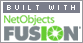Coming to Statistica
in 2016/2017

Group Classes

Interactive Webinars

Blog

Guestbook

.

E-mail        anne@statistica.com.au

# Place         Willetton, Western Australia# Mathematics & Statistic Tutor Perth - SPSS Help# Descriptive Statistics

Describing the data may be  in terms of pictures, numerical measures and probability.
We distinguish between populations and samples, Box plots (5 number summary calculations), Stem and Leaf plots, and Measures of Central Tendency and Variation.# Currently being updated but click to revisit the old material  apologies for the bad format and handwriting# Formula for Descriptive Statistics

Central Tendency and Variation have numerical measures that are coupled together. Mean and Standard Deviation are one, and Median and Interquartile Range are another.
Each couple are used when we have looked at the distribution.
We can transform are data adding and multiplying by numbers, this affects our measures.

# Normal Distributions

Normal distribution is a continuous distribution and one of the most commonly used one.  It will be come more important when we study Tests of Significance or Hypothesis Testing.
We will calculate a probability of being greater than or less than a number and the score or the number where so many percent is greater than or less than where you are given the percentage or probability.

# Central Limit Theorem

This is a very important theorem and will help with Normality assumptions.

# Flowchart for when to use z or t

This is a common problem in statistics units, you will need to pay attention to the population distribution, sample size and which standard deviation, population or sample.

# This is now Inferential Statistics and often students find this part difficult to cope with and apply to other situations.  So try this very basic explanation to  set you on to the path of statistical understanding.[Home] [Parents] [Contact us] [Services] [Mathematics] [Statistics] [School]